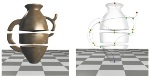## Computing Reeb graphs as a union of contour trees.Harish Doraiswamy and Vijay Natarajan.
IEEE Vis, Poster, 2011.

### Abstract

The Reeb graph of a scalar function tracks the evolution of the topology of its level sets. This work describes a fast and efficient algorithm to compute the Reeb graph of a scalar function defined over manifolds and non-manifolds. The key idea in the proposed approach is to maximize the use of the efficient contour tree algorithm to compute the Reeb graph. The algorithm proceeds by dividing the input into a set of simply connected interval volumes using the join tree of the scalar function and computes the Reeb graph by combining the contour trees of all the interval volumes. Since the key ingredient of this method is a series of union-find operations, the algorithm is fast in practice. The algorithm also extends to handle large data that do not fit in memory.